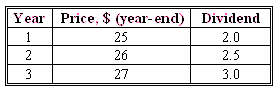### CFA Practice Question

There are 96 practice questions for this study session.

### CFA Practice Question

An investor is considering investing in a stock for between one year and three years. The company's stock is currently trading at \$24. He forecasts the following pattern of dividends and prices for the next 3 years:If the required rate of return from the stock is 12%, what is the maximum price that the investor should be willing to pay for this stock?
A. 24.11
B. 24.51
C. 25.13
Explanation: Since the investor is prepared to hold the stock for up to three years, we need to value this stock using one-, two- and three-period DDM:
• One-period DDM: V0 = D1 / (1 + r) + P1 / (1 + r) = 2 / (1 + 0.12) + 25 / (1 + 0.12) = \$24.11.
• Two-period DDM: V0 = D1 / (1 + r) + D2 / (1 + r)2 + P2 / (1 + r)2 = 2 / (1 + 0.12) + 2.5 / (1 + 0.12)2 + 26 / (1 + 0.12)2 = \$24.51.
• Three-period DDM: V0 = D1 / (1 + r) + D2 / (1 + r)2 + D3 / (1 + r)3 + P3 / (1 + r)3 = 2 / (1 + 0.12) + 2.5 / (1 + 0.12)2 + 3.0 / (1 + 0.12)3 + 27 / (1 + 0.12)3= \$25.13.
Hence the maximum value that the investor should be willing to pay for the stock is \$25.13.

User Comment
aero too much time to do it..... it won`t be possible on 4 june.
MGM13 Think again. This is exactly the kind of question they'd put on the exam, since it is designed to slow you down some.
danlan2 Use CF function on the calculator.
RNAN Yes, these are the "slow you down" type questions.
It is a good idea to learn how to use the CF function on the BAII Plus calculator. That way you can do these kind of questions real fast. These questions are easy points if you are fast but most people will just guess and move on. That means that most people will only get these right 25% of the time. That separates the people that pass from the people that fail.
volkovv I second RNAN with CF function take 20 seconds to solve it.
Xocrevilo With both price and dividends consistently rising, so value can be expected to increase over time: go straight for the 3-period DDM.
jingie careful xocrevilo, total return has to be greater than required return for your logic to work so I wouldn't use that as a rule of thumb
rana1970 all three prices are essentially the same in present time. as the question asks for max price, so it is C
endurance Use BAII, CF function, I=12% in all cases:

Period 1: CF0=0, CO1=2+25=27 ,NPV = 24.11
Period 2: CF0=0, CO1=2, CO2=2.5+26 =28.5 , NPV=24.51
Period 3: CF0=0, CO1=2, CO2=2,5 CO3=3+27=30, NPV=25.13